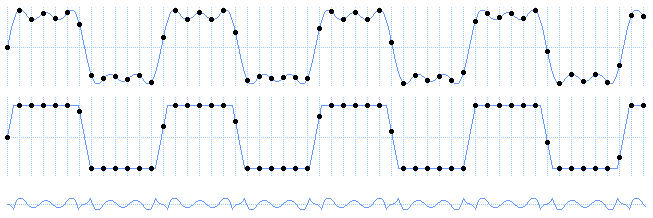# 12. Linear Interpolation Compared

Home | Next

A simple way of synthesizing waveforms like square waves is linear interpolation, where each output sample is determined by the average amplitude within the sample's time window. Synthesizing a square wave this way results in a single intermediate sample between amplitudes that matches the subsample offset of the transition.

Linear interpolation results in aliasing because averaging doesn't eliminate harmonics above the Nyquist limit. One way of seeing this is to compare a linear-interpolated square wave to a band-limited one.Band-limited square, linear-interpolated square, and difference (error)